Science Journal of Applied Mathematics and Statistics
Volume 4, Issue 3, June 2016, Pages: 115-118

Estimators Proposed by Geometric Mean, Harmonic Mean and Quadratic Mean

Vedat Sağlam, Tolga Zaman, Erdinç Yücesoy, Murat Sağır

Department of Statistics, Faculty of Science and Arts, Ondokuz Mayıs University, Kurupelit, Turkey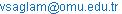(V. Sağlam)

Vedat Sağlam, Tolga Zaman, Erdinç Yücesoy, Murat Sağır, Estimators Proposed by Geometric Mean, Harmonic Mean and Quadratic Mean. Science Journal of Applied Mathematics and Statistics. Vol. 4, No. 3, 2016, pp. 115-118. doi: 10.11648/j.sjams.20160403.15

Received: May 16, 2016; Accepted: May 26, 2016; Published: June 7, 2016

Abstract: In the studies in literature up to date arithmetic population mean of auxiliary variable is used to obtain the proportional estimators. In this paper geometric mean, harmonic mean and quadratic mean is used as well as arithmetic population mean. Using geometric mean, harmonic mean and quadratic mean do not affect the variance of ratio estimator (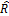). However new approaches are obtained for the estimation and variance of the dependent variable when these means are used as well as arithmetic population mean. In the application, the mean number of teaching staff of the departments in Ondokuz Mayıs University is estimated by auxiliary variable which is the number of students in the departments. In addition the variances of proportional estimation method are obtained and interpreted by using population arithmetic mean, geometric mean, harmonic and quadratic mean.

Keywords: Proportional Estimation, Geometric Mean, Harmonic Mean, Quadratic Mean, Inequalities

1. Introduction

One of the basic estimation method, using auxiliary variable to estimate the population mean, in simple random sampling is the proportional estimator method. In this study the proportional estimators, used to estimate population mean, and the variances of these estimators are given. In all studies regarding the proportional estimators, arithmetic mean is chosen as the population mean of the auxiliary variable. In this paper geometric mean, harmonic mean and quadratic mean are used as the population mean as well as arithmetic mean. Using these means as well as arithmetic will not change the variance of proportional estimator. However by using these means and under assumption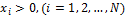, new approaches are obtained for the estimation of the dependent variable and its variance. Geometric mean, harmonic mean and quadratic mean are investigated because the population mean is fixed. The aim is to minimize the variance for the estimators. In the application part the data is obtained from the departments of Ondokuz Mayıs University. The number of teaching staff at the departments are estimated via the number of student which is considered as the auxiliary variable. Some other means of the population also calculated and variances are calculated for the proportional estimation method approaches. There are several new estimators in the literature proposed for population mean and population sum derived from known proportional estimation. "Cochran (1977) investigated the use of information obtained from the auxiliary variable ."

"Dayveh et al. (2003) proposed two estimators for the estimation of population mean using two auxiliary variable and generalized these estimators for more than two auxiliary variables ". "Sign and Tailor (2003) proposed new estimators by using correlation coefficient information ". "Ray and Singh (1981) developed a new proportional estimator using regression coefficient ". On the other hand "Kadılar and Çıngı (2004) investigated a special case of this estimator ". "Kadılar and Çıngı (2005) developed a new estimator, for population mean in two variable regression estimator, using the two auxiliary variable multiplicative estimator proposed by Dayveh et al. ". "İsaki (1983) proposed a proportional estimator for the population variance " and "by developing this estimator Prasad and Singh (1992) proposed new estimators for the population variance ". "Garcia and Cebrian (1996) proposed a new estimator for the population variance by using auxiliary variable and generalized this estimator for more than one auxiliary variable by a iteration method ". Proceeding from here the following estimators are proposed. "Beale (1962) and Tin (1965) used variation coefficients of dependent variable and independent variable as auxiliary variable to propose new proportional estimators for population mean [13,14]". Additionally "Zaman et al. (2014), investigated mean square errors of some ratio estimators and means via Taylor Series approach and made comparisons ". Proceeding from here the following estimators are proposed.

2. Proportional Estimators

Suppose that a sample with a sizeis chosen out of a population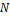by random simple sampling method. It is assumed that for the values of y and x the change of the proportion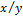is very small and the correlation between the variables is denoted by a line passing from the origin point. Then the sample means of the variables are as follows: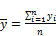(1)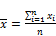(2)

The population means of the variables are: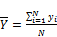(3)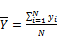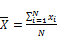(4)

If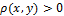then the proportional estimation of the population mean of variable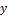is obtained as,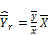(5)

and proportion of the variables is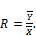(6)

The estimator of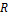is given as follows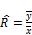(7)

Substituting equation (7) in equation (5), we have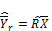(8)

"Cochran, 1977, ". The variance of the estimator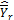is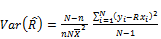(9)

"Yamane, 2001, "

3. Proportional Estimator and New Estimators Proposed by Other Means

New estimators are given by using geometric mean, harmonic mean and quadratic mean instead of arithmetic population mean in equation (8) under condition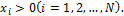3.1. Estimator Proposed by Geometric Mean

Definition 1. The geometric mean of a population is given as,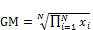(10)

where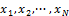are values of auxiliary variable of the population.

Replacing equation (10) instead of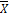in equation (8) we have following estimator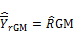(11)

Expected value of this estimator is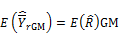(12)

or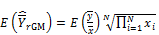(13)

and its variance is,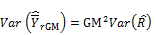(14)

or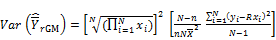(15)

3.2. Estimator Proposed by Harmonic Mean

Definition 2. The harmonic mean of a population is given as,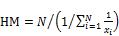(16)

Where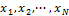are values of auxiliary variable of the population.

Replacing equation (16) instead of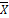in equation (8) the estimator,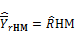(17)

is obtained.

Expected value of this estimator is,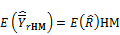(18)

or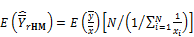(19)

and its variance is obtained as,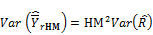(20)

or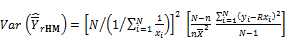(21)

3.3. Estimator Proposed by Quadratic Mean

Definition 3. The quadratic mean of a population is given as,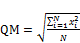(22)

whereare values of auxiliary variable of the population.

Replacing equation (22) instead ofin equation (8) the estimator,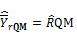(23)

is obtained.

Expected value of this estimator is,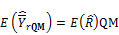(24)

or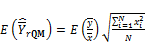(25)

and its variance is obtained as,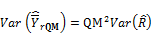(26)

or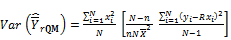(27)

4. Comparisons

4.1. The Comparison of the Estimators

The following inequalities are given for the comparison of arithmetic mean against other means "Shahbazov, 2005, "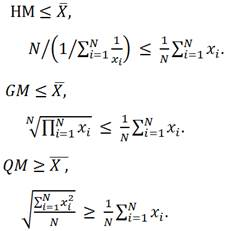(28)

whereare values of auxiliary variable of the population.

4.2. Comparison of the Means

Using inequalities (28), the following inequalities are obtained: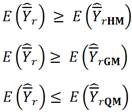(29)

4.3. The Comparison of the Variances

Similarly using inequalities (28), the inequalities for the variances of estimators are given below: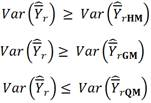(30)

In equation (30) the inequality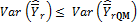is not a desirable situation therefore the estimatoris ignored. In this manner, using the following inequality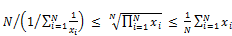(31)

we have,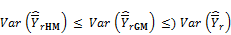(32)

According to these results, if the population values of the auxiliary variable are known and positive then the estimatorsor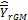can be proposed. An actual data application, presented in section 4, supports these results.

5. Application

The actual data needed for application is obtained from the actively teaching departments of Ondokuz Mayıs University. The number of teaching staff in the university is denoted by the variable y and the number of students in the university is denoted by the variable x. When the correlation between these two variables is positive the variable, considered by auxiliary variable, can be estimated. Each of the department in the university is population unit and the sample size estimation is calculated by equation (33) "Çıngı, 2009, "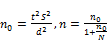(33)

In equation (33) when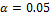,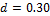the sample equals to 14. Arithmetic mean, geometric mean and harmonic mean of the number of students are given in Table 1 respectively.

Table 1. The population means of the variable.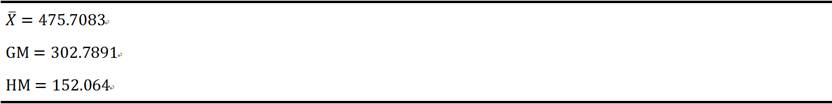Data of the chosen sample is obtained as in Table 2 by using random numbers table.

Table 2. Obtained values of the sample.

 Department Number of teaching staff Number of students Faculty of Medicine 290 1700 Fine Arts 11 439 Turkish Language 4 301 Foreign Languages 17 1164 Archaeology 5 204 Biology 23 262 Art History 3 196 Sociology 7 240 Economy 9 404 International Trade and Logistics 3 118 Communication 11 304 Chemistry Engineering 7 209 Music 9 28 Civil Aviation 11 189

The estimated values and estimated variances of the proposed new estimators are calculated by Table 2 and given in Table 3.

Table 3. The estimators and numerical values of the variances.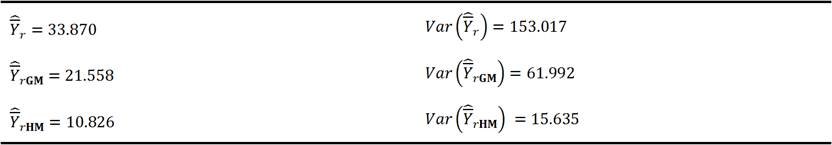In Table 3, we notice that the variances of estimators, obtained by using geometric and harmonic means, are smaller.

6. Conclusions

In this paper new mean estimators are proposed by the population geometric mean, population harmonic mean and population quadratic mean of the auxiliary variable. The expected values and variances of these proposed new estimators theoretically obtained. The estimators proposed by the quadratic mean are ignored since the variances of these estimators are greater than or equal to the variances of the estimators obtained by population arithmetic mean. However the estimators proposed by geometric mean and harmonic mean are analyzed since the variances of these estimators are smaller than or equal to the variances of estimators obtained by population arithmetic mean. When the obtained results are discussed it is seen that the variance of the estimator, proposed by harmonic mean, is the smallest. The aim of this paper, assuming that the population values of the auxiliary variable are positive, is to propose new estimators and to find their variances by using inequalities concerning the means. Finally an application is done using actual data to show that the numerical results tend to theoretically obtained results.

References

1. Cochran, W. G., "Sampling Techniques", John Wiley and Sons, New-York, 1977.
2. Dayyeh, W. A. A., Ahmed M. S., Ahmed R. A., Mutlak, H. A, "Some estimators of a finite population mean using auxiliary information", Applied Mathematics and Computation, 139, 287-298, 2003.
3. Singh, H. P. and Tailor, R., "Use of known correlation coefficient in estimating the finite population mean", Statistics in Transition, Vol. 6, No. 4, 555-560, 2003.
4. Ray, S. K. and Singh R. K., "Difference-cum-ratio type estimators, Journal of Indian Statistical Association", 19, 147- 151, 1981.
5. Kadılar, C. and Çıngı, H., "Ratio estimators in simple random sampling", Applied Mathematics and Computation, 151, 893-902, 2004.
6. Kadılar, C. and Çıngı, H, "A new estimator using two auxiliary variables", Applied Mathematics and Computation, 162, 901-908, 2005.
7. Yamane, T., "Temel Örnekleme Yöntemleri, (Çevirinler: Esin, Aydın, C. Bakır, M. A., Gürbüzsel, E.)" Literatür Yayınları, No: 53, İstanbul, 2001.
8. Shahbazov, A., "Introduction to Probability Theory", Birsen Yayınevi Ltd. Şti. Kod No: Y. 0029, ISBN: 975-511-415-7, İstanbul, 2005.
9. Çıngı, H., "Sampling Theory", H. Ü. Fen Fakültesi Basımevi, Beytepe, 2009.
10. Isaki, C. T., "Variance estimation using auxiliary information, Journal of American Statistical Assosiation", 78, 117-123, 1983.
11. Prasad, B., Singh, H. P., "Unbiased estimators of finite population variance using auxiliary information in sample surveys", 21, 5, 1367-1376, 1992.
12. Garcia, M. R., Cebrian, A. A., "Repeated subsitution method: The ratio estimator for the population variance", Metrika, 43, 101-105, 1996.
13. Beale, E. M. L., "Some use of computers in operational research, Industrielle Organisation", 31, 27-28, 1962.
14. Tin, M., "Comparison of some ratio estimators", Journal of American Statistical Assosiation, 60, 294-307, 1965.
15. Zaman, T., Sağlam, V., Sağir, M., Yücesoy, E., and Zobu, M., "Investigation of some estimators Taylor Series approach and an application", Science Publishing Group, 3 (5): 141-147, 2014.

 Contents 1. 2. 3. 3.1. 3.2. 3.3. 4. 4.1. 4.2. 4.3. 5. 6.
Article ToolsAbstractPDF(347K)